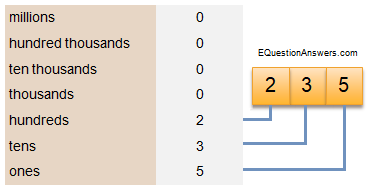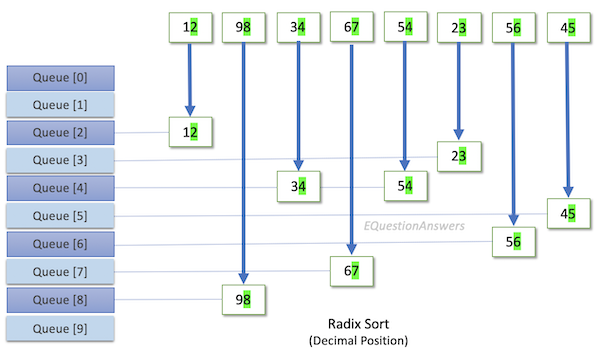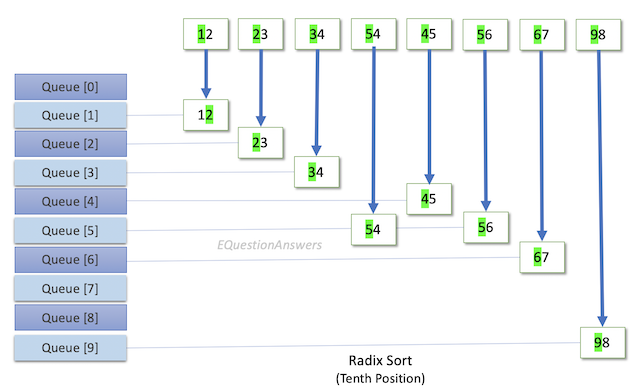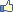This sorting algorithm is based on the values of the digits in the positional representation of numbers to be sorted.

## Numbers and decimal digits

Consider the number 235 in decimal notation It is written with 2 in the hundredth position, 3 in the tenth position and 5 in the units' position.To find which number is greater between two such numbers, it may be done in the following way: Starting with the least significant digit, check which number has larger digit. The number with the larger most significant digit is the greater number. If all digits match, the numbers are equal.

## Algorithm

This technique is implemented in radix sort algorithm.

The elements are populated in an array. The radix sort algorithm implements a 10 rows of queues. The queues are maintained corresponding to the elements in the decimal number system. Queue is for holding numbers whose digits ends with 0. Similarly queue to queue are for holding digits 1 - 9.

The algorithm takes each number from the array and extracts the digit from a specific position in the number. The digit is checked and put in the corresponding queue. Queue process starts with digit 0 till 9

Now all the queues are filled. It is time to dequeue the numbers and put those back to array. Dequeue is done in the same order, starting from zero till 9. Now the array is repopulated with numbers based on the rank of the digit position.

This process is repeated starting from least significant digit to most significant digit. Once the above process completes for the the most significant digit, it completes the sorting process.

Let us consider the following example: Considering the following numbers:

`	12	98	34	67	54	23	56	45`

The algorithm creates the following two queue sets:

Queue based on least significant digit:```Radix sort digit position ones
Queue  :
Queue  :
Queue  : 12,
Queue  : 23,
Queue  : 34, 54,
Queue  : 45,
Queue  : 56,
Queue  : 67,
Queue  : 98,
Queue  :
```

After repopulating array:

`	12	23	34	54	45	56	67	98`

Since the highest number in the array (98) has two digits, the process is repeated for the next digit, i.e. the most significant digit.

Queue based on most significant digit:```Radix sort digit position tens
Queue  :
Queue  : 12,
Queue  : 23,
Queue  : 34,
Queue  : 45,
Queue  : 54, 56,
Queue  : 67,
Queue  :
Queue  :
Queue  : 98,
```

After repopulating array:

`	12	23	34	45	54	56	67	98`

Thus, the array is sorted.

## Extract Digits

The method responsible for extraction of the digit from a particular position may be indicated by GetDigitatPosition(int number, int position) where number sent for extraction and position from which digit has to be extracted.

The algorithm for the method may be written as:

 int GetDigitatPosition(int number, int position) { int digit; while (position > 0) { digit = number % 10; number = number / 10; position--; } return digit; }

The method implementing radix sort may be defined as radix_sort(int elements[], int count) where elements[] is the array containing the elements, count is the number of elements present in the array. This function calculates the highest number and the digits present in that number.

It performs mainly these functions in a loop:

• The numbers are provided to the GetDigitatPosition(int number, int position) method along with position for digit to be extracted
• The number is put in the queue pointed by the extracted digit
• The elements in the queues are put back into the array after all the numbers have been checked.

These steps are repeated from digits in zero th position till the highest digit in the max number present in the array.

The algorithm for the method may be written as:

 void RadixSort(int elements[], int count) { /* 10 queues for sorting digits */ queue  *q; int i, j, k, digits, max = -2147483648; /* Determine max number */ for (i = 0; i < count; i++) { if (elements[i] > max) { max = elements[i]; } } i = 0; /* Determine max decimal digits */ while (max) { max = max /10; i++; } digits = i; /* Take elements from digit = 0 to max digit and sort */ for (i = 0; i < digits; i++) { /* Initialize queue */ for (int j=0; j < 10; j++) { q[j] = new queue; } /* Populate the queue(s) based on digit */ for ( k=0; k < count; k++) { /* get the digit */ int digit = GetDigitatPosition(elements[k], i+1); /* enqueue at the rare of digit's queue */ q[digit]->push(elements[k]); } /* Dequeue individual queues to elements array */ k = 0; for ( j=0; j < 10; j++) { while (!q[j]->empty()) { /* dequeue from front */ elements[k] = q[j]->front(); q[j]->pop(); k++; } } /* Cleanup the queues */ for ( j=0; j < 10; j++) { delete q[j]; } } }

## Time complexities

The time requirement for the radix sorting method depends on the number of digits and the number of elements in the array (count). Since the outer loop for(i= 0;i< digits;i++) is traversed "digits" times and inner loop is traversed "count" times, the sort is approximately O(max digits*count). this sort is reasonably efficient when the number of digits in the numbers is small.

## Source code

Full working code of radix sort written in C language however C++ STL queue class has been utilized for simplicity.

 /* Radix sort CPP file */ #include  #include  #include  using namespace std; int array[]={2000,300, 256, 12, 98, 34, 67, 54, 23, 56, 45}; char * radix_positios [] = { "ones", "tens", "hundreds", "thousands", "ten thousands", "hundred thousands", "millions" }; /* get the digit at position */ int GetDigitatPosition(int number, int position) { int digit; while (position > 0) { digit = number % 10; number = number / 10; position--; } return digit; } /* print the entire number array */ void PrintEntrieArray(int elements[], int count) { printf("Element :"); for (int index = 0; index < count; index ++) { printf("%d, ",elements[index]); } printf("\n"); } /* Radix sort algorithm */ void RadixSort(int elements[], int count) { /* 10 queues for sorting digits */ queue  *q; int i, j, k, max = -2147483648; /* Determine max number */ for (i = 0; i < count; i++) { if (elements[i] > max) { max = elements[i]; } } i = 0; /* Determine max decimal digits */ while (max) { max = max /10; i++; } max = i; /* Take elements from digit = 0 to max digit and sort */ for (i = 0; i < max; i++) { printf("Radix sort position %s\n", (i < 7 )? radix_positios[i] : ""); /* Initialize queue */ for (int j=0; j < 10; j++) { q[j] = new queue; } /* Populate the queue(s) based on digit */ for ( k=0; k < count; k++) { /* get the digit */ int digit = GetDigitatPosition(elements[k], i+1); /* enqueue at the rare of digit's queue */ q[digit]->push(elements[k]); } /* Dequeue individual queues to elements array */ k = 0; for ( j=0; j < 10; j++) { printf("Queue [%d] : ", j); while (!q[j]->empty()) { /* dequeue from front */ elements[k] = q[j]->front(); q[j]->pop(); printf("%d, ", elements[k]); k++; } printf("\n"); } /* Cleanup the queues */ for ( j=0; j < 10; j++) { delete q[j]; } PrintEntrieArray(elements, count); } } int main(int argc, char* argv[]) { /* print unsorted array */ printf("Radix sort\n"); PrintEntrieArray(array, 11); /* run radix sort */ RadixSort(array, 11); /* print sorted array */ printf("Radix sorted array\n"); PrintEntrieArray(array, 11); return 0; }

## Output

```Radix sort
Element :12, 98, 34, 67, 54, 23, 56, 45,
Queue  :
Queue  :
Queue  : 12,
Queue  : 23,
Queue  : 34, 54,
Queue  : 45,
Queue  : 56,
Queue  : 67,
Queue  : 98,
Queue  :
Element :12, 23, 34, 54, 45, 56, 67, 98,
Queue  :
Queue  : 12,
Queue  : 23,
Queue  : 34,
Queue  : 45,
Queue  : 54, 56,
Queue  : 67,
Queue  :
Queue  :
Queue  : 98,
Element :12, 23, 34, 45, 54, 56, 67, 98,
Element :12, 23, 34, 45, 54, 56, 67, 98,
Press any key to continue
```

## Output

```Radix sort
Element :2000, 300, 256, 12, 98, 34, 67, 54, 23, 56, 45,
Queue  : 2000, 300,
Queue  :
Queue  : 12,
Queue  : 23,
Queue  : 34, 54,
Queue  : 45,
Queue  : 256, 56,
Queue  : 67,
Queue  : 98,
Queue  :
Element :2000, 300, 12, 23, 34, 54, 45, 256, 56, 67, 98,
Queue  : 2000, 300,
Queue  : 12,
Queue  : 23,
Queue  : 34,
Queue  : 45,
Queue  : 54, 256, 56,
Queue  : 67,
Queue  :
Queue  :
Queue  : 98,
Element :2000, 300, 12, 23, 34, 45, 54, 256, 56, 67, 98,
Queue  : 2000, 12, 23, 34, 45, 54, 56, 67, 98,
Queue  :
Queue  : 256,
Queue  : 300,
Queue  :
Queue  :
Queue  :
Queue  :
Queue  :
Queue  :
Element :2000, 12, 23, 34, 45, 54, 56, 67, 98, 256, 300,
Queue  : 12, 23, 34, 45, 54, 56, 67, 98, 256, 300,
Queue  :
Queue  : 2000,
Queue  :
Queue  :
Queue  :
Queue  :
Queue  :
Queue  :
Queue  :
Element :12, 23, 34, 45, 54, 56, 67, 98, 256, 300, 2000,
Element :12, 23, 34, 45, 54, 56, 67, 98, 256, 300, 2000,
Press any key to continue
```

You have viewed 1 page out of 248. Your C learning is 0.00% complete. Login to check your learning progress.0

## Recomemded books from Amazon